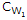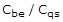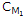# Electronic Devices - BJT and FET Frequency Response

### Exercise :: BJT and FET Frequency Response - General Questions

31.

Which of the following capacitors is (are) included in Ci for the high-frequency region of a BJT or FET amplifier?

 A. Input wiring capacitanceB. The transition capacitance () C. Miller capacitanceD. All of the above

Explanation:

No answer description available for this question. Let us discuss.

32.

In the input RC circuit of a single-stage BJT, by how much does the base voltage lead the input voltage for frequencies much larger than the cutoff frequency in the low-frequency region?

 A. About 0º B. 45º C. About 90º D. None of the above

Explanation:

No answer description available for this question. Let us discuss.

33.

In the ________-frequency region, the capacitive elements of importance are the interelectrode (between terminals) capacitances internal to the active device and the wiring capacitance between the leads of the network.

 A. low B. mid C. high

Explanation:

No answer description available for this question. Let us discuss.

34.

What is the ratio of the output power to the input power at the cutoff frequencies in a normalized frequency response plot?

 A. 0.25 B. 0.5 C. 0.707 D. 1